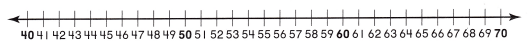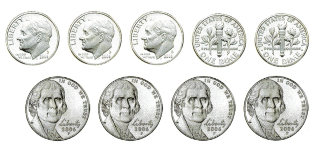Refer to our Texas Go Math Grade 2 Answer Key Pdf to score good marks in the exams. Test yourself by practicing the problems from Texas Go Math Grade 2 Unit 2 Assessment Answer Key.

Concepts and Skills

Break apart the numbers you are subtracting. Write the difference. (TEKS 2.4.B)Question 1.
65 – 7 = _________
65 – 7 = 58
Explanation:
7 is written as 5 and 2
65 – 5 = 60
60 – 2 = 58

Question 2.
62 – 18 = _____________
62 – 18 = 44
Explanation:
18 is written as
2 + 10 + 6
62 – 2 = 60
60 – 10 = 50
50 – 6 = 44

Write a story problem that could be shown with the number sentence. Then solve. (TEKS 2.4.D)

Question 3.
32 – 17 = ☐
32 – 17 = 15
Explanation:
A farm has 32 hens out of it 17 are red
and remaining are white
how many white hens are in the farm
15 hens are in the farm

Use the pictures to describe equal groups. (TEKS 2.6.A)

Question 4.There are __________ groups of buttons.
Each group has __________.
So, there are _____________ in all.
There are 5 groups of buttons.
Each group has 3.
So, there are 15 in all.
Explanation:
3 + 3 + 3+ 3+ 3  = 15
5 x 3 = 15

Fill in the bubble for the correct answer choice.

Question 5.
What is the total value of these coins? (TEKS 2.5.A)(A) 60¢
(B) 40¢
(C) 70¢
Explanation:
1 quarter = 25
1 dime = 10
2 x 25 = 50
2 x 10 = 20

Question 6.
Alex had 43 stickers. He gave 19 stickers to his sister. How many stickers does Alex have now? (TEKS 2.4.B)(A) 24
(B) 34
(C) 26
Explanation:
He gave 19 stickers to his sister.
43 – 19 = 24
24 stickers that Alex have now

Question 7.
Bianca has 35 ribbons. Kim has 24 red ribbons and 23 blue ribbons. How many more ribbons does Kim have than Bianca? (TEKS 2.4.C)(A) 11
(B) 12
(C) 9
Explanation:
Bianca has 35 ribbons.
Kim has 24 red ribbons and
23 blue ribbons.
24 + 23 = 47
47 – 35 = 12
12 more ribbons that Kim have than Bianca

Question 8.
362 children are at school. 171 of the children are boys. How many of the children are girls? (TEKS 2.4.C)
(A) 191
(B) 183
(C) 211
Explanation:
362 children are at school.
171 of the children are boys.
362 – 171 = 191
191 of the children are girls

Question 9.
There are 10 bags with 2 marbles in each bag. There are 4 boxes with 10 marbles in each box. How many marbles are there? (TEKS 2.6.A)(A) 34
(B) 60
(C) 26
Explanation:
There are 10 bags with 2 marbles in each bag.
There are 4 boxes with 10 marbles in each box.
10 x 2 = 20
4 x 10 = 40
40 + 20 = 60
60 marbles are there

Question 10.
There are 3 plates with 5 grapes on each plate. Which number sentence shows how to find the number of grapes that are on the plates? (TEKS 2.6.A)(A) 5 + 5 + 5 = 15
(B) 3 + 5 = 8
(C) 3 + 5 + 3 = 11
Explanation:
There are 3 plates with 5 grapes on each plate.
5 + 5 + 5 = 15 number sentence shows how to find the number of grapes that are on the plates

Question 11.
Nelson has these coins. What other coins does he need to have one dollar in all? (TEKS 2.5.A)(A) 3 nickels
(B) 3quarters
(C) dimes
Explanation:
1 dime = 10 cents
5 x 10 = 50
1 nickel = 5 cents
4 x 5 = 20
50 + 20 = 70
3 dimes are needed

Question 12.
There are 24 children in Sam’s class. 4 children are sitting at each table in the classroom. At how many tables are the children sitting? (TEKS 2.6.B)
(A) 20
(B) 6
(C) 28
Explanation:
There are 24 children in Sam’s class.
4 children are sitting at each table in the classroom.
24 ÷ 4 = 6
At 6 tables the children are sitting

Question 13.
There are 245 melons on the truck and 172 melons in the store. How many melons are there altogether? (TEKS 2.4.C)
(A) 417
(B) 73
(C) 427
Explanation:
There are 245 melons on the truck
and 172 melons in the store.
245 + 172 = 417
417 melons are there altogether

Fill in the bubble for the correct answer choice.

Question 14.
Mr. Blakely has 158 peaches at his store. 84 peaches are on a table. 33 peaches are in a basket. The rest of the peaches are in bags. How many peaches are in bags? (TEKS 2.4.C)(A) 41
(B) 107
(C) 51
Explanation:
Mr. Blakely has 158 peaches at his store.
84 peaches are on a table.
33 peaches are in a basket.
84 + 33 = 117
The rest of the peaches are in bags.
158 – 117 = 41
41 peaches are in bags

Question 15.
Steve has 3 climes, 6 nickels, and 10 pennies. What is the total value of Steve’s coins? (TEKS 2.5.A, 2.5.B)
total value: ____________
Circle coins below to make another group of coins that have the same total value. Cross out the coins that you do not use.Explain how you decided which coins to use for the new group of coins. (TEKS 2.5.A)
1 dime = 10 cents
3 x 10 = 30
1 nickel = 5 cents
6 x 5 = 30 cents
10 pennies = 10 cents
30 + 30 + 10 = 70 cents
Explanation:
The total value of Steve’s coins is 70 cents
For the new group of coins we used 2 quarter coins
2 x 25 = 50
2 dimes
2 x 10 = 20

Scroll to Top